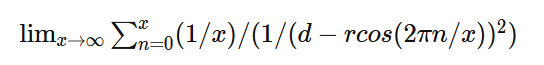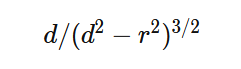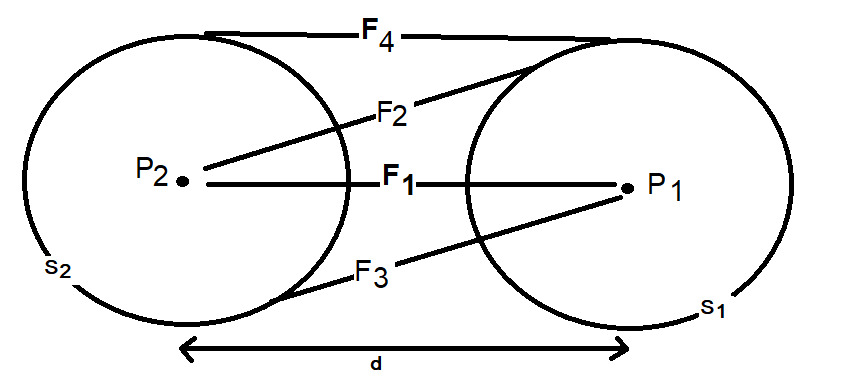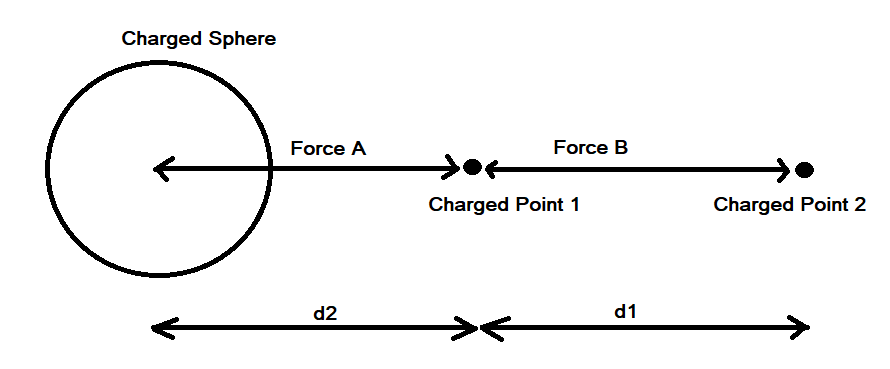# Simple Unification Theory

## Recommended Posts

Hi,

Completely new to the forum.  Please be kind.  Just looking for some feedback on an idea that a single equation to calculate both the force of molecular bonds and the force of gravity is possible if a sphere is not approximated as a point at the molecular level using conventional Coulombic forces.

Anyway, my attempt at explaining it is here https://newtontweaked.com/

Colin.

##### Share on other sites

Hi. Welcome to the forums. Please, take a look at Section 2 point 7 of the guidelines.

Quote

Links, pictures and videos in posts should be relevant to the discussion, and members should be able to participate in the discussion without clicking any links or watching any videos. Videos and pictures should be accompanied by enough text to set the tone for the discussion, and should not be posted alone.

A sphere is never "approximated by a point" when calculating gravitational forces outside the sphere. It's just that the field of both objects happens to be the same. That's called Newton's theorem, and in the case of General Relativity, Birkhoff's theorem. A different case would be if the matter distribution were not spherically symmetric.

Molecular bonds are a completely different matter, and you really need some quantum mechanics to tackle them...

##### Share on other sites

On 10/10/2021 at 11:01 PM, joigus said:

Hi. Welcome to the forums. Please, take a look at Section 2 point 7 of the guidelines.

!

Moderator Note

Thanks joigus! Let's make this official. ColinJ, can you copy/paste from your document and post it here? It's important to make sure each step in an idea is sound before taking another.

##### Share on other sites

Apologies for the delay – was on the road for a few days.

The entire thing is a strange concept.  My argument is that a simple numerical approximation using a spreadsheet disagrees with the shell theorem.  Below is the approach for the numerical approximation.  Also attached is a spreadsheet implementing the diagram.The numerical approximation in the spreadsheet over n rows is shown as a Riemann sum below.Which evaluates toIf you use that equation, and not the classic q1q2/r^2 equation, for point to sphere interaction a Hydrogen molecule now has four different electrostatic forces at play, well three in the case of Hydrogen gas.And the weird part - if you graph the net force over distance of all forces you get a Lennard Jones curve.

And the peak is about where it should be at stable molecular bond distance and the tail trails off infinitely with an ever decreasing attractive force ( a bit like gravity).

Lots of people have looked at this and said it cannot be correct but nobody can tell me what is actually incorrect other than it disagrees with pretty much everything physics is based on.

Cheers,

Colin.##### Share on other sites

6 hours ago, ColinJ said:

If you use that equation, and not the classic q1q2/r^2 equation

I'm confused. Are you saying your equation is not derived using the Coulomb force equation?

##### Share on other sites

It is confusing.  I'm saying that the Coulombic force of q1q2/r^2 does not apply between a point and a hollow sphere.  Although conventionally a force calculation between a point and a hollow sphere is approximated as a point and another point and q1q2/r^2 is used.  But, if you run a very simple numerical approximation between a point and a hollow sphere where the sphere is represented as n points, using the standard Coulombic equation between the n points and sum the forces it does not equal the force of q1q2/r^2 when the sphere is represented as point.Assuming distances d1 and d2 are identical and the charges on points 1, 2 and the sphere are identical the electrostatic force using Coulomb’s Law between points 1 and 2 does not equal the electrostatic force between point 1 and the charged sphere.

##### Share on other sites

Is nobody replying because I haven't explained this properly or because this concept means a lot of physics is wrong?  I am reminded of Monty Python - I came here for an argumentBut, I could be arguing my spare time.

## Create an account

Register a new account